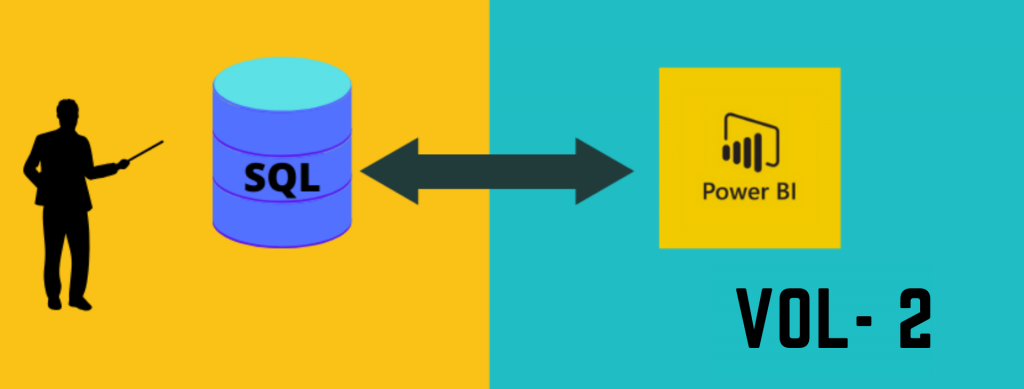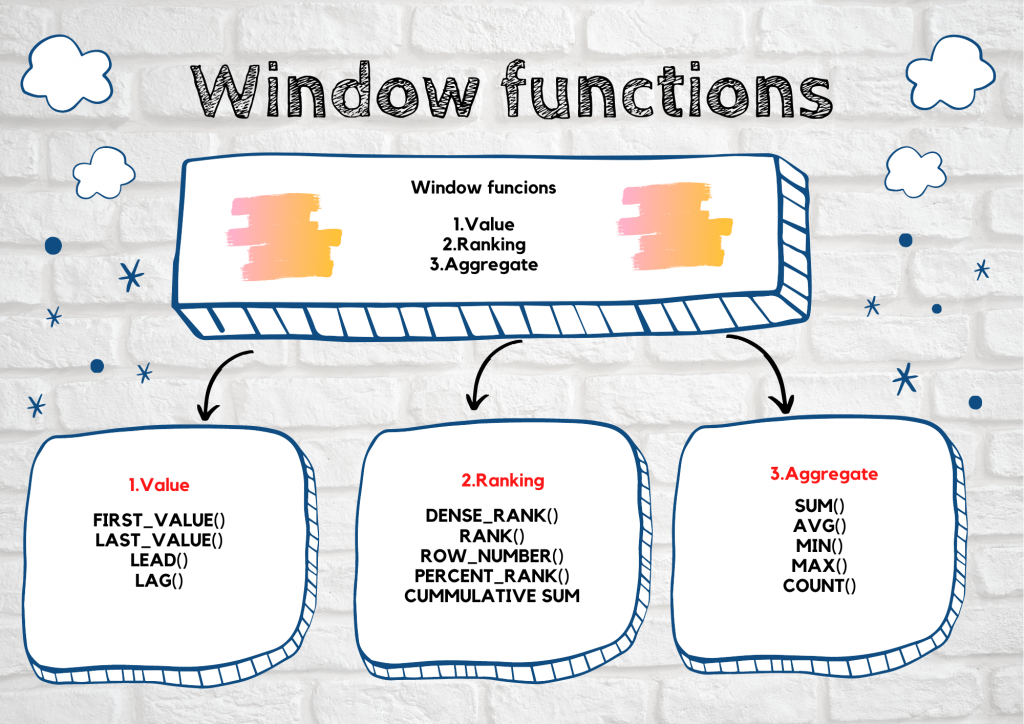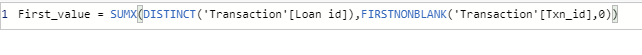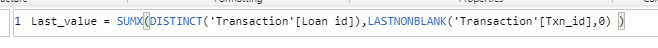# SQL equivalent Power BI DAX functions – Part 2## Introduction

In this article, we are going to see window function in SQL and its equivalent Power BI DAX function.If you want to check out SQL basic, check it out in part 1.

### Specifications

• Data – Loan transaction data
• Environment – Postgres and Power BI

## What is window function?

A window function performs an aggregate calculation across a set of table rows and returns single or multiple rows based on the function used with it.

## Window function classification

Window function comprises many different functions and it is grouped into 3 different categories.### Value

#### First_value

The first_value() function is a window function that returns the first value in a group or within the group.

#### Last_value

The last_value() function is also a window function that returns the last value in a group or within the group.

Both the functions are implemented in Power BI by using SUMX, FIRSTNONBLANK, LASTNONBLANK.

Example;

For each loan, the first and last transaction made is find by using these two functions. Its equivalent Power BI function also is given below.Lead is a window function that is used to fetch the next row value in the current row value itself.

#### Lag

Lag is also a window function that is used to retrieve the previous row value in the current row value itself.

In Power BI, it is not easy to obtain lead and lag value for any record.

But lag function can be obtained by the PREVIOUS function in Power BI where the date should be involved.click here to know more

Even lead function can be obtained by creating the index and adding 1 to index to obtain the value. click here to know more

### Ranking

#### Rank

The Rank() function is a window function that assigns rank within the group or multiple groups. If two or more rows have the same rank it will skip the next rank.

#### Dense rank

The Dense_rank() function is also a window function similar to rank() function but it will not skip rank when two or more rows have the same ranks.

Both the rank functions can be implemented in Power BI DAX by using the RANKX() function with the parameter passed.

#### Row number

Rownum function is used to create the row number in different ways like within a group or multiple groups.

The row number generated by using the CALCULATE function in  Power BI.

Example:

In here, I explain the row number created within loan number ordering on the transaction-id

#### Percentile rank

Percentile_rank() is a window function that returns the percentage of scores in its frequency distribution that is equal or lower than that.

It is calculated in Power BI by using the CALCULATE, RANKX, and DIVIDE functions.

Example:

Percentile rank within loan id based on the transaction.

#### Cumulative sum

Cumulative sum refers to running sum and it is implemented in SQL by using sum with a window function and in Power BI implemented by using CALCULATE and SUM function.

Example:

Running sum of transaction amount within the loan id.

### Aggregate

Windows function acts on the aggregate functions and returns the output by row-level known as window aggregate function. The same can be obtained in Power BI by using the formatting option available at the field level.

Example:

Here I explain the aggregate function on the transaction records by the customer level.

Some of the aggregate functions are:

#### SUM

Return the sum of the transaction amount.

#### AVG

Return the average value of the transaction amount

#### MIN

Return the minimum of the transaction amount.

#### MAX

Return the maximum of the transaction amount.

#### COUNT

Return count of records of the transaction.

Windows aggregate function will be faster than normal group by function in SQL. So these are the some of the window function equivalent in Power BI.

## Author

•Tableau Certified Data Analytics professional with 7+ years of overall IT experience and 4 years of experience in analytics. Completed Post graduate program in Data science and Engineering from Great Lakes. Having good knowledge in Tableau, PowerBI, SQL and Python. Member of Data Visualization Society(DVS). Currently working as Data Analyst in Elsevier, a publishing and information analytics company.

### 3 thoughts on “SQL equivalent Power BI DAX functions – Part 2”

1.Hello,

thanks for these 2 articles! They’re really amazing for someone who is trying to do some DAX coding after years of SQL experience. Can you please clarify about ROW_NUMBER() equivalent one moment:
– SQL let’s you define OVER(PARTITION BY …) – how to do this in DAX? All your examples show calculations only for one ID.

Thanks,
Danil

1.1. Row_number function I used selected data. If I remove the filter on one ID the row number order will be based on the transaction id ascending order.
2. Cumulative function does the same OVER(PARTITION BY …). the only difference is the cumulative sum of each transaction partition by loan id.
If you don’t want cumulative just remove the last expression txn_id

2.Hi,
Could you please let me know how to achieve the functionality in Dax without using Earlier for
the sum() over(partition by col1 order by col2 ASC ROWS BETWEEN CURRENT ROW AND 3 FOLLOWING)
Thanks,
Sandeep